# S-shaped curves

## 2004-02-12

Competitive binding experiments will often need a nonlinear regression model. This model has to level off at both extremes to represent almost no binding at one end and saturated binding at the other end. This behavior is usually represented by an S-shaped curve. In this web page, I will describe some of the equations that you might use to represent an S-shaped curve.

A very simple S-shaped curve.

A very simple formula that serves as a starting point isHere is a graph of this function.Stretching and shrinking the S-shaped curve

Multiplying x by a constant,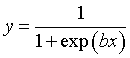yields S shaped curves that are either shrunk towards the center or stretched out.A negative value of b,yields curves that rise rather than fall.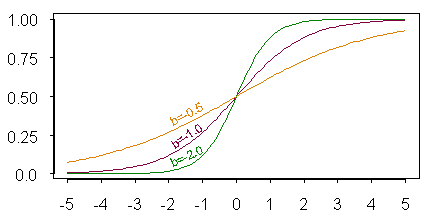Shifting the S-shaped curve

You can subtract a constant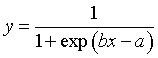to shift the curve.Negative values for a, of course, would shift the curve to the left.

Selecting starting values

When you fit an S shaped curve to your data, you need to provide some reasonable starting values. They don’t have to be perfect, but they do have to be reasonable. Here’s some general guidance.

The parameter “b” represents how spread out the data. When b=1, the middle 50% of the curve (0.25 to 0.75) will span a little more than 2 units on the x-axis. So divide 2 by the estimated span of the middle 50% in your graph and use that as a starting value for b. Also keep in mind that a negative value for b represents an S-shaped curve which increases rather than decreases.

The ratio a/b represents the value where the S-shaped curve reaches 0.5, the midway point on the curve. Sometimes this midpoint is called the IC50 because it is the concentration that inhibits 50% of the binding.

If you have a rough idea what the IC50 would be (either from previous studies or an estimate off the line graph), you would multiply this estimate by the starting value that you selected for b to get a starting value for a.

First example

Here’s some data from a normalized assay of competitive binding. X is the concentration in log10 units, and yn is the normalized signal.

x      yn


-6.0 0.02 -6.3 0.10 -6.6 0.29 -7.0 0.20 -7.3 0.80 -7.6 1.00

Here is a graph of the data.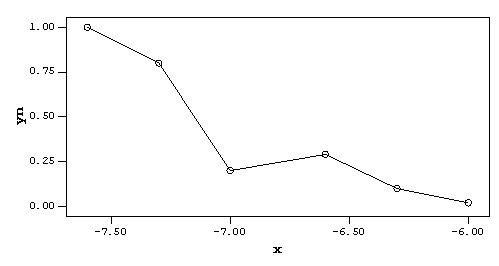The middle 50% ranges from about -7.3 to -7.1. So a reasonable starting values b is 2 / 0.2 = 10. The midway point looks like it is close to -7.2. Setting the ratio a/b equal to -7.2, you should get -72 as a starting value for a.

SPSS dialog boxes

Select ANALYZE | REGRESSION | NONLINEAR from the SPSS menu.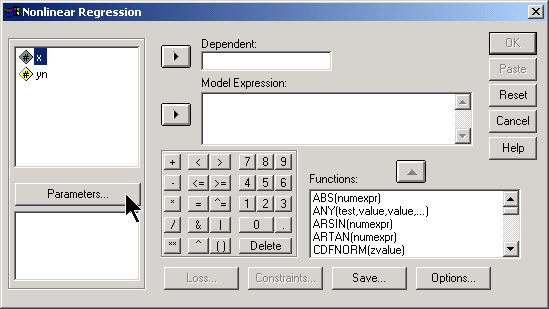First, click on the parameters button.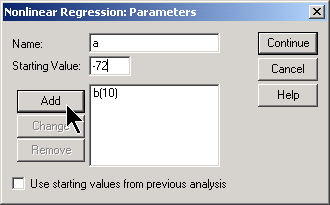Specify b and a as parameters with starting values of 10 and -72, respectively. Then click on the CONTINUE button.

Now include yn as the dependent variable and specify the formula for the equation as 1 / (1 + exp (b * x - a)).Then click on the OK button.

SPSS output

This produces a lot of output. Since the output is so large, you need to double click on it to get a scroll bar. Here’s the top third of the output.Non-linear regression uses an interative estimation approach, and SPSS provides you with details of these iterations. The residual SS is a measure of how good the estimates are: it starts at 0.10 and declines to 0.09 as the estimates improve. Our values of b and a decline slightly as the model improves.The remaining iterations appear in the middle third of the screen. You should examine the reason why iterations stopped. Here they stopped because the improvements in Residual SS became too small–at the last step, for example, the residual SS improved only in the ninth significant digit. This reason for stopping is usually a good sign.

Also notice the Nonlinear Regression Summary Statistics, which is actually an analysis of variance table. The Residual SS at the final step appears here in a rounded form (.08982). Notice that the residual SS is small relative to the Uncorrected Total and the Corrected Total. This is a sign that your model fits the data reasonably well.

SPSS also produces an R squared value. The interpretation of R squared is dependent, at times, on the particular nonlinear regression model. As a general rule, though, an R squared value close to 1.0 is an indication that the model fits the data well.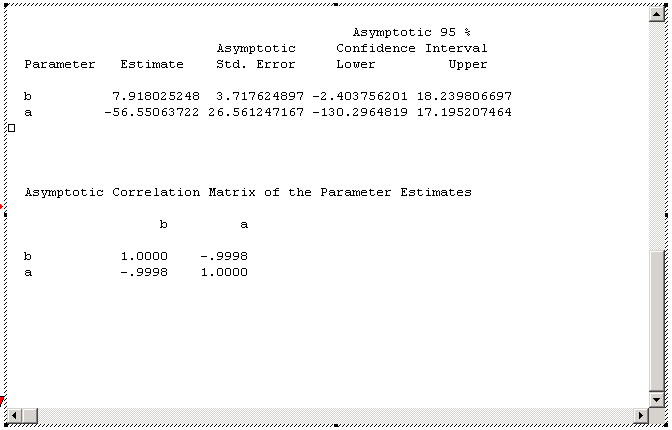The bottom third of the output gives parameter estimates, confidence intervals, and correlations among the parameters. The ratio of a/b gives an estimate of the IC50 (-56.55 / 7.92 = 7.1).

Predicted values

You can get predicted values for the regression equation by clicking on the SAVE button. If you add some extra x values with missing yn values, you can also get predictions at some of the intermediate points. A plot of the predicted values shows the general shape of the S-shaped curve.Notice that this curve badly misses on two of the values (x = -6.3, and x = -6.6), but comes very close to the remaining four data values. There is no way, of course to get an S-shaped curve to come close to all the data when the data has an extra “hump” so perhaps this is as good as we can get.

Poorly specified starting values

I often have difficulty with nonlinear regression. Many times it is because I chose very bad starting values. To illustrate what can go wrong, I deliberately chose unusual starting values.

In the first example, I chose a starting value of -10 rather than +10 for b. This is easy to do if you forget whether the S-shaped curve is rising or falling.Notice how the values for b and a hop madly about. This is a sign of bad starting values, but it looks like SPSS finally settled down on reasonable values for a and b. Unfortunately, when you plot the predicted values, you see an S-shaped curve that doesn’t come anywhere close to your data.The curve you see is just a small fragment of the S-shaped curve, with most of the interesting portions extending off well beyond the range of the data.

As another example of what can go wrong, suppose I naively set the starting value for a to 0. Here’s what the output looks like.There are three warning signs here. First the model stopped at the first iteration. Second, it stopped for an unusual reason. Third, the model has negative sum of squares.

The remainder of the output also has some warning signs.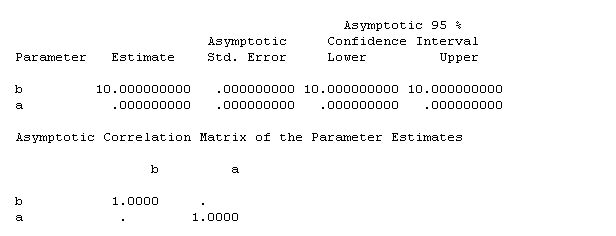Notice that the two parameters have zero standard errors, which almost never happens with real data. Finally the correlation between b and a is missing.

Alternative models

Go back and look at the model with good starting values. Even here, there are some issues. Notice how wide the confidence intervals are in spite of this being a reasonably good fit to the data. Also notice how strongly correlated the two parameter estimates are. Both of these things are signs of trouble. What is happening is that the parameters are so highly correlated that it is difficult to estimate either one individually with any precision.

There are two ways to fix this. First, some (but not all) nonlinear regression models work better if you center the independent variable(s). You center a variable by subtracting its mean, so that the center of the data is now around zero. Select ANALYZE | DESCRIPTIVE STATISTICS | DESCRIPTIVES to find out that the mean of X is -6.8. Then select TRANSFORM | COMPUTE from the SPSS menu to create a new variable, XC, which is X - (-6.8).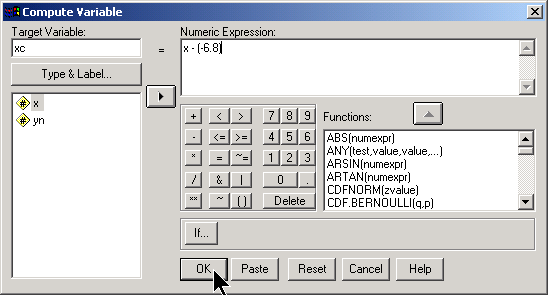Here’s what the centered data looks like.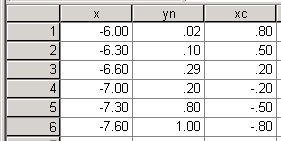Fit the same nonlinear regression model, with two changes:

1. The variable “xc” replaces x, and
2. The starting value for a is now -4 = (-7.2 - (-6.8)) * 10.

Here are the results.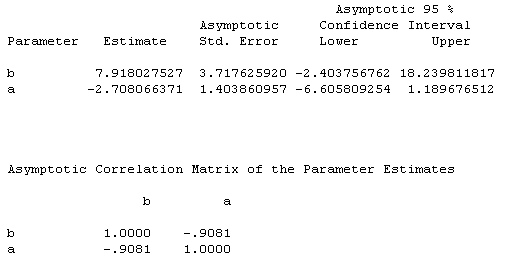There is some improvement–the correlation is down to -0.9081 from -0.9998, but the confidence intervals are still rather wide.

The estimate of the IC50 is about the same, as long as we remember to add back the mean (-6.8 + (-2.71) / 7.92 = -7.1).

A second approach is to reparameterize the nonlinear formula. By “reparameterize”, I mean provide a formula that looks a bit different because some of the parameters have been re-arranged. Here is a reparameterization that is worth considering:Here is the output.Notice that the parameters u and b are almost perfectly uncorrelated. The confidence interval for b is still wide, but the confidence interval for u is narrower than the confidence interval for a would have been. Perhaps this is comparing apples to oranges, but I believe that the reparameterized model is more useful.

One aspect of this reparameterized model is that u, rather than the ratio a/b, is a direct estimate of the IC50. We can get confidence limits for the IC50 as well (-7.3 to -6.9).

S-shaped curves with arbitrary upper and lower bounds

In this experiment, a positive and negative control were used to set the ceiling and floor of the S-shaped curve. But perhaps these controls were themselves subject to random error, so it might be interesting to allow the nonlinear regression model to set the upper and lower limits rather than arbitrarily fixing them at the control levels.

Here’s a plot of the data with the positive and negative controls. In theory, the controls represent the limits at plus or minus infinity, but I just set them well outside the range of the remaining data.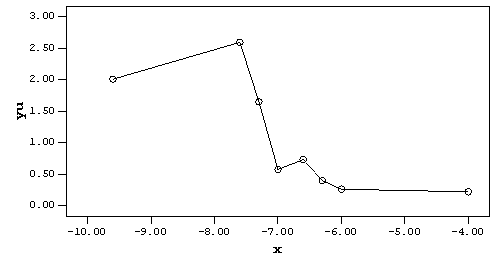Notice how the signal at x = -7.6 extends above the ceiling defined by the negative control. When we normalized this data, we truncated that value at 1.0 since the simple S-shaped curve could not extend above 1.0 or below 0.0. With the raw or unnormalized data, we can allow the model itself to set the ceiling, possibly at a value slightly larger than the negative control and slightly smaller than the signal at -7.6.

The formula for the S-shaped curve adds two additional parameters, c and d.The parameter c represents the floor, the minimum value of the S-shaped curve, and the curve rises to a maximum value of c+d. For starting values, we can set c to the positive control level (0.2) and if we set c+d to the negative control level (2.0) then a starting value of 1.8 for d seems reasonable. Here’s the output.The estimated floor and ceiling levels are a bit surprising. The floor (c) is 0.4, which is larger than the positive control and the ceiling (c+d) is 2.3, which is larger than the negative control. This model has an IC50 which is slightly smaller (-7.2) than what the earlier model had computed (-7.1).

Here’s a graph of the fitted curve.This graph confirms the observations we noted above. Notice how the curve levels off at a value well above the positive control and well about the signal at x = -6.0.

Comparing two S-shaped curves

Often in competitive binding assays, we want to compare the IC50 for different proteins. Using nonlinear regression, we can fit a pair of parallel S-shaped curves to each protein.

x     in    y


-6.0 0 0.02 -6.3 0 0.10 -6.6 0 0.29 -7.0 0 0.20 -7.3 0 0.80 -7.6 0 1.00 -6.0 1 0.00 -6.3 1 0.18 -6.6 1 0.33 -7.0 1 0.27 -7.3 1 1.00 -7.6 1 1.00

The middle column is an indicator variable which equals zero for the first protein and one for the second protein. Here’s a plot of the data.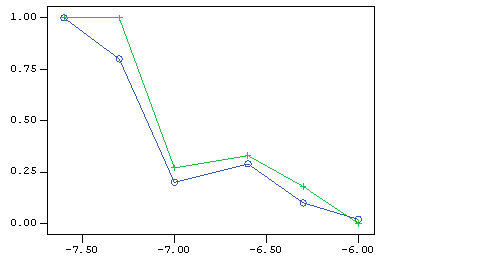The blue circles represent the first protein. The green pluses represent the second protein.

To compare the two proteins we will incorporate the indicator variable into the nonlinear regression model and add a parameter to estimate the difference between the two S-shaped curves.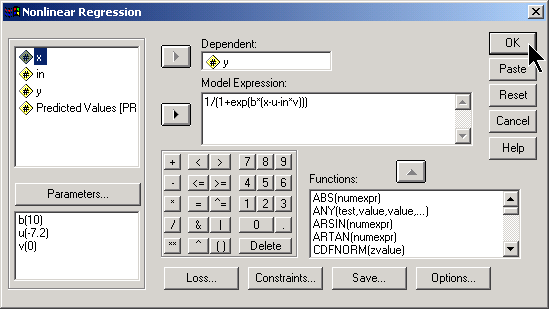Here is the output.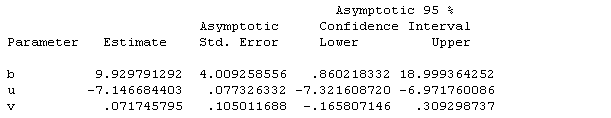The estimate for u (-7.1) is the estimated IC50 for the first protein. The estimate for v (0.07) is the difference in the IC50 between the two proteins. The 95% confidence interval for v goes from -0.17 to 0.31, which indicates that the estimated difference could easily result just from sampling error.

Here is a graph of the data.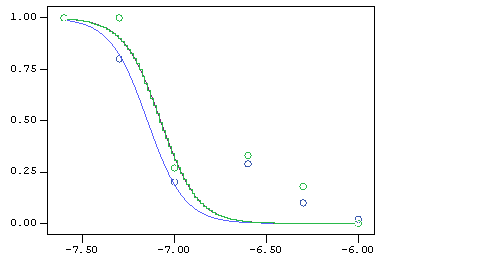There is a slight separation between the two S-shaped curves. Notice also that both proteins show a small “hump” at x =-6.6 and -6.3 which neither S-shaped curve can fit well. This should be investigated further.

You can find an earlier version of this page on my original website.